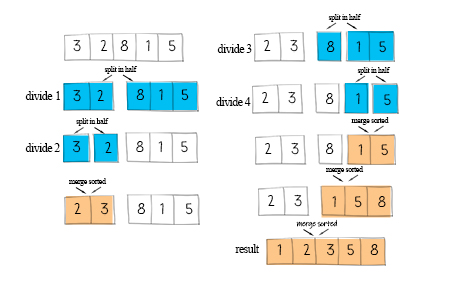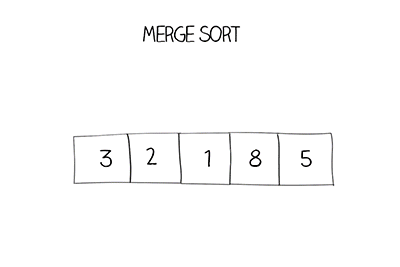Merge sort uses divide and conquer technique to provide fast and stable sorting. Divide-and-conquer is a technique works by recursively breaking down a problem into two or more sub-problems of the same or related type, until these become simple enough to be solved directly.### How merge sort works?

It divides the array in half recursively until to single item. Then merges two sorted arrays until all elements are sorted.

### What is the time complexity of merge sort?

Merge sort operates in O(nlogn) time. It is faster than simple sorting such as bubble sort, selection sort and insertion sort. The space complexity is O(n) because it uses an extra array when merge.### Merge sort Implementation

The merge method is to compare each element in two sorted array one by one, put the smaller one in the third array until one of arrays reaches the end. Then copy the rest of unfinished array to the third array. This requires an additional array in memory, equal in size to the one being sorted.

Merge sort is an efficient sorting technique. It has O(nlogn) complexity. The downside is that it requires extra space for the additional array. The space complexity is O(n).